# The Universe of Discourse

Fri, 29 Jul 2016

As I have mentioned before, I am not a sudden-flash-of-insight person. Every once in a while it happens, but usually my thinking style is to minutely examine a large mass of examples and then gradually synthesize some conclusion about them. I am a penetrating but slow thinker. But there have been a few occasions in my life when the solution to a problem struck me suddenly out of the blue.

One such occasion was on the first day of my sophomore honors physics class in 1987. This was one of the best classes I took in my college career. It was given by Professor Stephen Nettel, and it was about resonance phenomena. I love when a course has a single overarching theme and proceeds to examine it in detail; that is all too rare. I deeply regret leaving my copy of the course notes in a restaurant in 1995.

The course was very difficult, But also very satisfying. It was also somewhat hair-raising, because of Professor Nettel's habit of saying, all through the second half “Don't worry if it doesn't seem to make any sense, it will all come together for you during the final exam.” This was not reassuring. But he was right! It did all come together during the final exam.

The exam had two sets of problems. The problems on the left side of the exam paper concerned some mechanical system, I think a rod fixed at one end and free at the other, or something like that. This set of problems asked us to calculate the resonant frequency of the rod, its rate of damping at various driving frequencies, and related matters. The right-hand problems were about an electrical system involving a resistor, capacitor, and inductor. The questions were the same, and the answers were formally identical, differing only in the details: on the left, the answers involved length, mass and stiffness of the rod, and on the right, the resistance, capacitance, and inductance of the electrical components. It was a brilliant exam, and I have never learned so much about a subject during the final exam.

Anyway, I digress. After the first class, we were assigned homework. One of the problems was

Show that every function is the sum of an even function and an odd function.

(Maybe I should explain that an even function is one which is symmetric across the !!y!!-axis; formally it is a function !!f!! for which !!f(x) = f(-x)!! for every !!x!!. For example, the function !!x^2-4!!, shown below left. An odd function is one which is symmetric under a half-turn about the origin; formally it satisfies !!f(x) = -f(-x)!! for all !!x!!. For example !!\frac{x^3}{20}!!, shown below right.)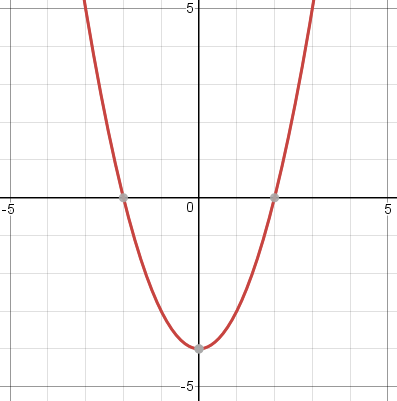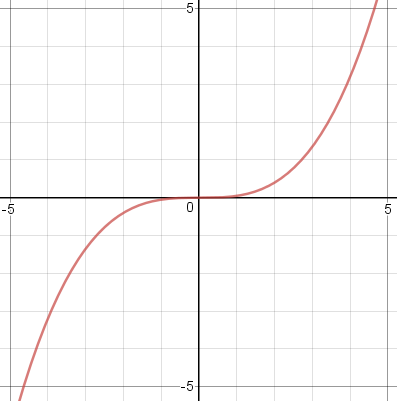I found this claim very surprising, and we had no idea how to solve it. Well, not quite no idea: I knew that functions could be expanded in Fourier series, as the sum of a sine series and a cosine series, and the sine part was odd while the cosine part was even. But this seemed like a bigger hammer than was required, particularly since new sophomores were not expected to know about Fourier series.

I had the privilege to be in that class with Ron Buckmire, and I remember we stood outside the class building in the autumn sunshine and discussed the problem. I might have been thinking that perhaps there was some way to replace the negative part of !!f!! with a reflected copy of the positive part to make an even function, and maybe that !!f(x) + f(-x)!! was always even, when I was hit from the blue with the solution:

\begin{align} f_e(x) & = \frac{f(x) + f(-x)}2 \text{ is even},\\ f_o(x) & = \frac{f(x) - f(-x)}2 \text{ is odd, and}\\ f(x) &= f_e(x) + f_o(x) \end{align}

So that was that problem solved. I don't remember the other three problems in that day's homework, but I have remembered that one ever since.

But for some reason, it didn't occur to me until today to think about what those functions actually looked like. Of course, if !!f!! itself is even, then !!f_e = f!! and !!f_o = 0!!, and similarly if !!f!! is odd. But most functions are neither even nor odd.

For example, consider the function !!2^x!!, which is neither even nor odd. Then we get

\begin{align} f_e(x) & = \frac{2^x + 2^{-x}}2\\ f_o(x) & = \frac{2^x - 2^{-x}}2 \end{align}

The graph is below left. The solid red line is !!2^x!!, and the blue and purple dotted lines are !!f_e!! and !!f_o!!. The red line is the sum of the blue and purple lines. I thought this was very interesting-looking, but a little later I realized that I had already known what these graphs would look like, because !!2^x!! is just like !!e^x!!, and for !!e^x!! the even and odd components are exactly the familiar !!\cosh!! and !!\sinh!! functions. (Below left, !!2^x!!; below right, !!e^x!!.)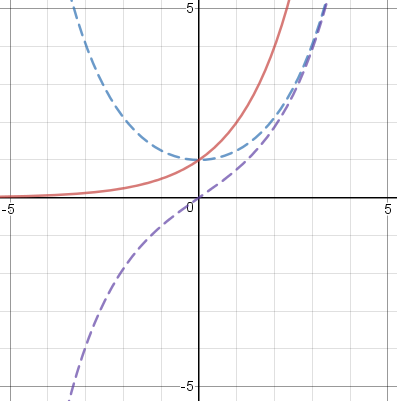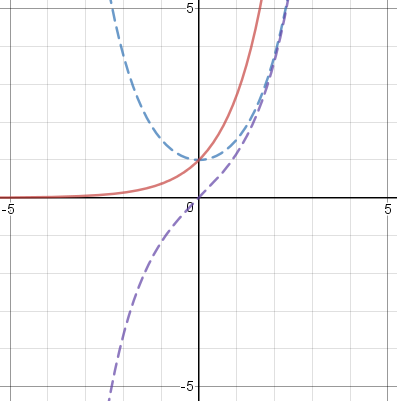I wasn't expecting polynomials to be more interesting, but they were. (Polynomials whose terms are all odd powers of !!x!!, such as !!x^{13} - 4x^5 + x!!, are always odd functions, and similarly polynomials whose terms are all even powers of !!x!! are even functions.) For example, consider !!(x-1)^2!!, which is neither even nor odd. We don't even need the !!f_e!! and !!f_o!! formulas to separate this into even and odd parts: just expand !!(x-1)^2!! as !!x^2 - 2x + 1!! and separate it into odd and even powers, !!-2x!! and !!x^2 + 1!!: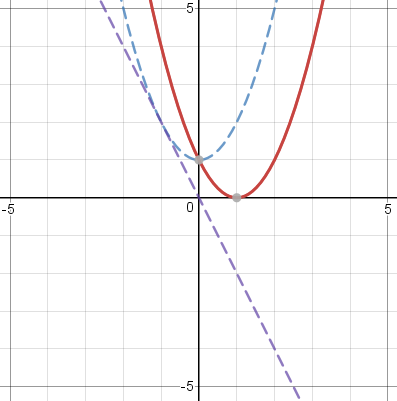Or we could do !!\frac{(x-1)^3}3!! similarly, expanding it as !!\frac{x^3}3 - x^2 + x -\frac13!! and separating this into !!-x^2 -\frac13!! and !!\frac{x^3}3 + x!!: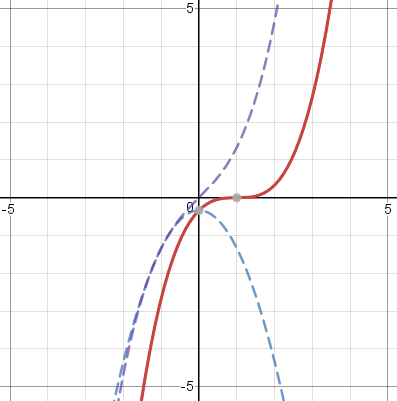I love looking at these and seeing how the even blue line and the odd purple line conspire together to make whatever red line I want.

I kept wanting to try familiar simple functions, like !!\frac1x!!, but many of these are either even or odd, and so are uninteresting for this application. But you can make an even or an odd function into a neither-even-nor-odd function just by translating it horizontally, which you do by replacing !!x!! with !!x-c!!. So the next function I tried was !!\frac1{x+1}!!, which is the translation of !!\frac 1x!!. Here I got a surprise. I knew that !!\frac1{x+1}!! was undefined at !!x=-1!!, so I graphed it only for !!x>-1!!. But the even component is !!\frac12\left(\frac1{1+x}+\frac1{1-x}\right)!!, which is undefined at both !!x=-1!! and at !!x=+1!!. Similarly the odd component is undefined at two points. So the !!f = f_o + f_e!! formula does not work quite correctly, failing to produce the correct value at !!x=1!!, even though !!f!! is defined there. In general, if !!f!! is undefined at some !!x=c!!, then the decomposition into even and odd components fails at !!x=-c!! as well. The limit $$\lim_{x\to -c} f(x) = \lim_{x\to -c} \left(f_o(x) + f_e(x)\right)$$ does hold, however. The graph below shows the decomposition of !!\frac1{x+1}!!.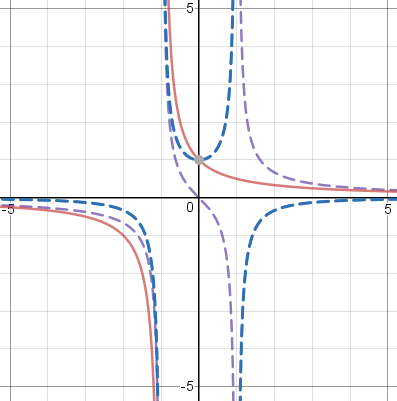Vertical translations are uninteresting: they leave !!f_o!! unchanged and translate !!f_e!! by the same amount, as you can verify algebraically or just by thinking about it.

Following the same strategy I tried a cosine wave. The evenness of the cosine function is one of its principal properties, so I translated it and used !!\cos (x+1)!!. The graph below is actually for !!5\cos(x+1)!! to prevent the details from being too compressed: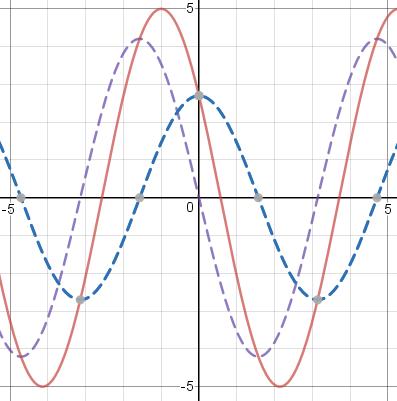This reminded me of the time I was fourteen and graphed !!\sin x + \cos x!! and was surprised to see that it was another perfect sinusoid. But I realized that there was a simple way to understand this. I already knew that !!\cos(x + y) = \sin x\cos y + \sin y \cos x!!. If you take !!y=\frac\pi4!! and multiply the whole thing by !!\sqrt 2!!, you get $$\sqrt2\cos\left(x + \frac\pi4\right) = \sqrt2\sin x\cos\frac\pi4 + \sqrt2\cos x\sin\frac\pi4 = \sin x + \cos x$$ so that !!\sin x + \cos x!! is just a shifted, scaled cosine curve. The decomposition of !!\cos(x+1)!! is even simpler because you can work forward instead of backward and find that !!\cos(x+1) = \sin x\cos 1 + \cos x \sin 1!!, and the first term is odd while the second term is even, so that !!\cos(x+1)!! decomposes as a sum of an even and an odd sinusoid as you see in the graph above.

Finally, I tried a Poisson distribution, which is highly asymmetric. The formula for the Poisson distribution is !!\frac{\lambda^xe^\lambda}{x!}!!, for some constant !!\lambda!!. The !!x! !! in the denominator is only defined for non-negative integer !!x!!, but you can extend it to fractional and negative !!x!! in the usual way by using !!\Gamma(x+1)!! instead, where !!\Gamma!! is the Gamma function. The !!\Gamma!! function is undefined at zero and negative integers, but fortunately what we need here is the reciprocal gamma function !!\frac1{\Gamma(x)}!!, which is perfectly well-behaved. The results are spectacular. The graph below has !!\lambda = 0.8!!.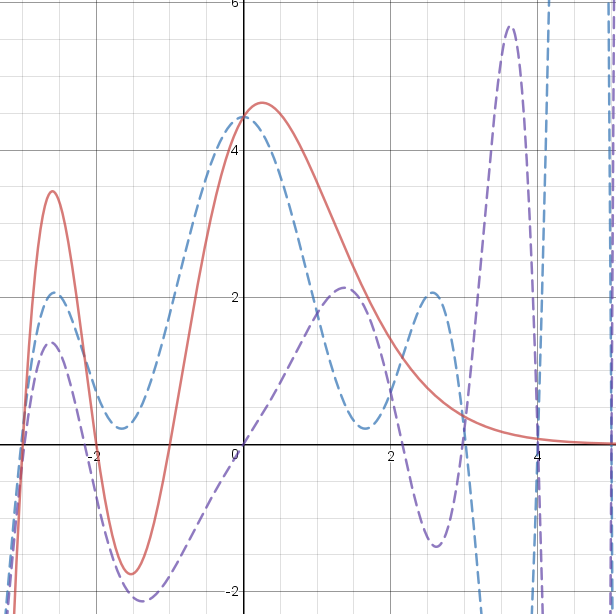The part of this with !!x\ge 0!! is the most interesting to me, because the Poisson distribution has a very distinctive shape, and once again I like seeing the blue and purple !!\Gamma!! functions working together to make it. I think it's just great how the red line goes gently to zero as !!x!! increases, even though the even and the odd components are going wild. (!!x! !! increases rapidly with !!x!!, so the reciprocal !!\Gamma!! function goes rapidly to zero. But the even and odd components also have a !!\frac1{\Gamma(-x)}!! part, and this is what dominates the blue and purple lines when !!x >4!!.)

On the !!x\lt 0!! side it has no meaning for me, and it's just wiggly lines. It hadn't occurred to me before that you could extend the Poisson distribution function to negative !!x!!, and I still can't imagine what it could mean, but I suppose why not. Probably some statistician could explain to me what the Poisson distribution is about when !!x<0!!.

You can also consider the function !!\sqrt x!!, which breaks down completely, because either !!\sqrt x!! or !!\sqrt{-x}!! is undefined except when !!x=0!!. So the claim that every function is the sum of an even and an odd function fails here too. Except perhaps not! You could probably consider the extension of the square root function to the complex plane, and take one of its branches, and I suppose it works out just fine. The geometric interpretation of evenness and oddness are very different, of course, and you can't really draw the graphs unless you have four-dimensional vision.

I have no particular point to make, except maybe that math is fun, even elementary math (or perhaps especially elementary math) and it's fun to see how it works out.

The beautiful graphs in this article were made with Desmos. I had dreaded having to illustrate my article with graphs from Gnuplot (ugh) or Wolfram|α (double ugh) and was thrilled to find such a handsome alternative.

[ Addendum: I've just discovered that in Desmos you can include a parameter in the functions that it graphs, and attach the parameter to a slider. So for example you can arrange to have it display !!(x+k)^3!! or !!e^{-(x+k)^2}!!, with the value of !!k!! controlled by the slider, and have the graph move left and right on the plane as you adjust the slider, with its even and odd parts changing in real time to match. ]

[ For example, check out travelling Gaussians or varying sinusoid. ]